## Section 4.1: Angles

### Learning Outcomes

• Draw angles in standard position.
• Convert between degrees and radians.
• Convert between decimal degrees and degrees, minutes, seconds
• Find coterminal angles.
• Find the length of a circular arc.
• Find the area of a sector of a circle.
• Use linear and angular speed to describe motion on a circular path.

A golfer swings to hit a ball over a sand trap and onto the green. An airline pilot maneuvers a plane toward a narrow runway. A dress designer creates the latest fashion. What do they all have in common? They all work with angles, and so do all of us at one time or another. Sometimes we need to measure angles exactly with instruments. Other times we estimate them or judge them by eye. Either way, the proper angle can make the difference between success and failure in many undertakings. In this section, we will examine properties of angles.

## Draw angles in standard position

Properly defining an angle first requires that we define a ray. A ray consists of one point on a line and all points extending in one direction from that point. The first point is called the endpoint of the ray. We can refer to a specific ray by stating its endpoint and any other point on it. The ray in Figure 1 can be named as ray EF, or in symbolic form $\overrightarrow{EF}$.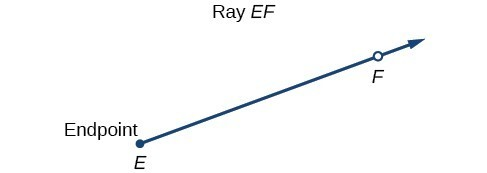Figure 1

An angle is the union of two rays having a common endpoint. The endpoint is called the vertex of the angle, and the two rays are the sides of the angle. The angle in Figure 2 is formed from $\overrightarrow{ED}$ and $\overrightarrow{EF}$. Angles can be named using a point on each ray and the vertex, such as angle ${DEF}$, or in symbol form $\angle{DEF}$.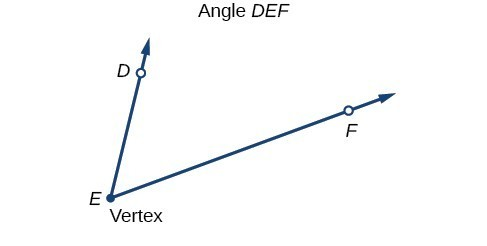Figure 2

Greek letters are often used as variables for the measure of an angle. The table below is a list of Greek letters commonly used to represent angles, and a sample angle is shown in Figure 2.

 $\theta$ $\phi \text{ or }\varphi$ $\alpha$ $\beta$ $\gamma$ theta phi alpha beta gamma

Angle creation is a dynamic process. We start with two rays lying on top of one another. We leave one fixed in place, and rotate the other. The fixed ray is the initial side, and the rotated ray is the terminal side. In order to identify the different sides, we indicate the rotation with a small arc and arrow close to the vertex as in Figure 4.

The following video provides an illustration of angles in standard position.

As we discussed at the beginning of the section, there are many applications for angles, but in order to use them correctly, we must be able to measure them. The measure of an angle is the amount of rotation from the initial side to the terminal side. Probably the most familiar unit of angle measurement is the degree. One degree is $\frac{1}{360}$ of a circular rotation, so a complete circular rotation contains 360 degrees. An angle measured in degrees should always include the unit “degrees” after the number, or include the degree symbol °. For example, 90 degrees = 90°.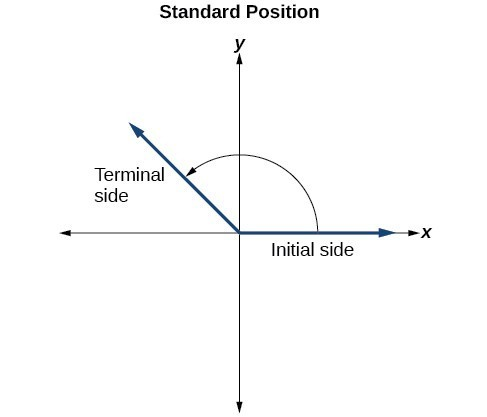Figure 5

To formalize our work, we will begin by drawing angles on an xy coordinate plane. Angles can occur in any position on the coordinate plane, but for the purpose of comparison, the convention is to illustrate them in the same position whenever possible. An angle is in standard position if its vertex is located at the origin, and its initial side extends along the positive x-axis.

If the angle is measured in a counterclockwise direction from the initial side to the terminal side, the angle is said to be a positive angle. If the angle is measured in a clockwise direction, the angle is said to be a negative angle.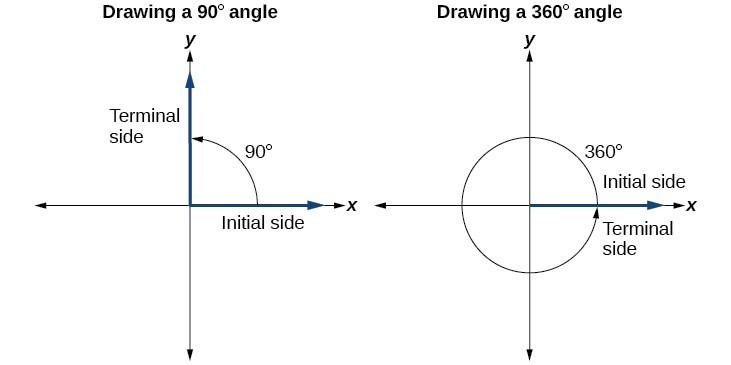Figure 6

Drawing an angle in standard position always starts the same way—draw the initial side along the positive x-axis. To place the terminal side of the angle, we must calculate the fraction of a full rotation the angle represents. We do that by dividing the angle measure in degrees by 360°. For example, to draw a 90° angle, we calculate that $\frac{90^\circ }{360^\circ }=\frac{1}{4}$. So, the terminal side will be one-fourth of the way around the circle, moving counterclockwise from the positive x-axis. To draw a 360° angle, we calculate that $\frac{360^\circ }{360^\circ }=1$. So the terminal side will be 1 complete rotation around the circle, moving counterclockwise from the positive x-axis. In this case, the initial side and the terminal side overlap.

Since we define an angle in standard position by its terminal side, we have a special type of angle whose terminal side lies on an axis, a quadrantal angle. This type of angle can have a measure of 0°, 90°, 180°, 270° or 360°.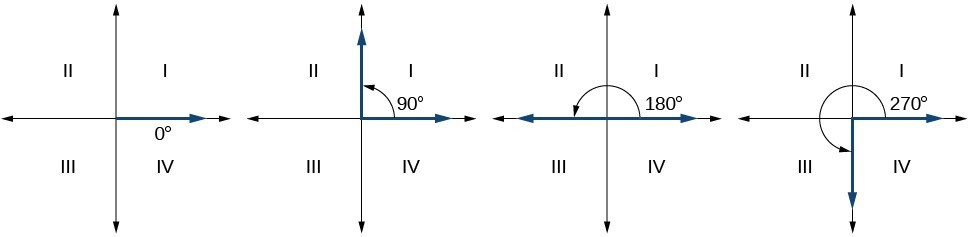Figure 7. Quadrantal angles have a terminal side that lies along an axis. Examples are shown.

### A GENERAL NOTE: QUADRANTAL ANGLES

Quadrantal angles are angles whose terminal side lies on an axis, including 0°, 90°, 180°, 270°, or 360°.

### HOW TO: GIVEN AN ANGLE MEASURE IN DEGREES, DRAW THE ANGLE IN STANDARD POSITION.

1. Express the angle measure as a fraction of 360°.
2. Reduce the fraction to simplest form.
3. Draw an angle that contains that same fraction of the circle, beginning on the positive x-axis and moving counterclockwise for positive angles and clockwise for negative angles.

### EXAMPLE 1: DRAWING AN ANGLE IN STANDARD POSITION MEASURED IN DEGREES

1. Sketch an angle of 30° in standard position.
2. Sketch an angle of −135° in standard position.

### TRY IT

Show an angle of 240° on a circle in standard position.

### Try It

Watch this video for more examples of determining angles of rotation.

## Converting Between Degrees and Radians

Dividing a circle into 360 parts is an arbitrary choice, although it creates the familiar degree measurement. We may choose other ways to divide a circle. To find another unit, think of the process of drawing a circle. Imagine that you stop before the circle is completed. The portion that you drew is referred to as an arc. An arc may be a portion of a full circle, a full circle, or more than a full circle, represented by more than one full rotation. The length of the arc around an entire circle is called the circumference of that circle.

The circumference of a circle is $C=2\pi r$. If we divide both sides of this equation by $r$, we create the ratio of the circumference to the radius, which is always $2\pi$ regardless of the length of the radius. So the circumference of any circle is $2\pi \approx 6.28$ times the length of the radius. That means that if we took a string as long as the radius and used it to measure consecutive lengths around the circumference, there would be room for six full string-lengths and a little more than a quarter of a seventh, as shown in Figure 10.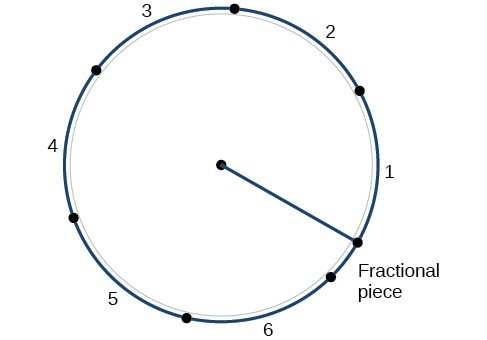Figure 10

This brings us to our new angle measure. One radian is the measure of a central angle of a circle that intercepts an arc equal in length to the radius of that circle. A central angle is an angle formed at the center of a circle by two radii. Because the total circumference equals $2\pi$ times the radius, a full circular rotation is $2\pi$ radians. So

$\begin{gathered} 2\pi \text{ radians}={360}^{\circ } \\ \pi \text{ radians}=\frac{{360}^{\circ }}{2}={180}^{\circ } \\ 1\text{ radian}=\frac{{180}^{\circ }}{\pi }\approx {57.3}^{\circ } \end{gathered}$

Note that when an angle is described without a specific unit, it refers to radian measure. For example, an angle measure of 3 indicates 3 radians. In fact, radian measure is dimensionless, since it is the quotient of a length (circumference) divided by a length (radius) and the length units cancel out.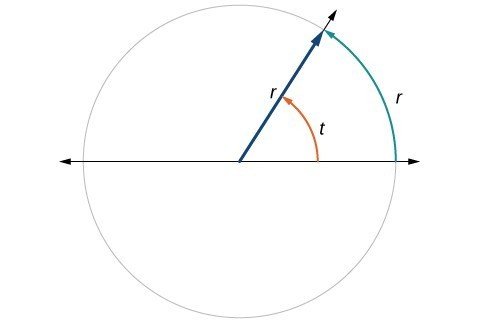Figure 11. The angle t sweeps out a measure of one radian. Note that the length of the intercepted arc is the same as the length of the radius of the circle.

## Relating Arc Lengths to Radius

An arc length $s$ is the length of the curve along the arc. Just as the full circumference of a circle always has a constant ratio to the radius, the arc length produced by any given angle also has a constant relation to the radius, regardless of the length of the radius.

This ratio, called the radian measure, is the same regardless of the radius of the circle—it depends only on the angle. This property allows us to define a measure of any angle as the ratio of the arc length $s$ to the radius $r$.

$\begin{gathered}s=r\theta \\ \theta =\frac{s}{r}\end{gathered}$

If $s=r$, then $\theta =\frac{r}{r}=\text{ 1 radian}\text{.}$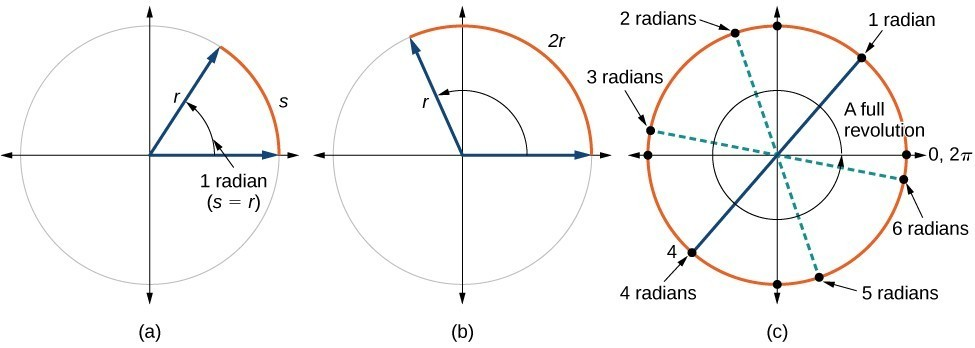Figure 12. (a) In an angle of 1 radian, the arc length $s$ equals the radius $r$. (b) An angle of 2 radians has an arc length $s=2r$. (c) A full revolution is $2\pi$ or about 6.28 radians.

To elaborate on this idea, consider two circles, one with radius 2 and the other with radius 3. Recall the circumference of a circle is $C=2\pi r$, where $r$ is the radius. The smaller circle then has circumference $2\pi \left(2\right)=4\pi$ and the larger has circumference $2\pi \left(3\right)=6\pi$. Now we draw a 45° angle on the two circles, as in Figure 13.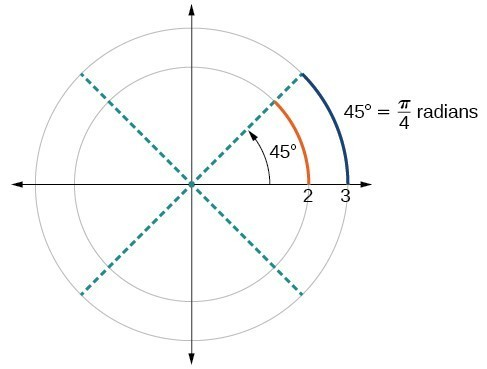Figure 13. A 45° angle contains one-eighth of the circumference of a circle, regardless of the radius.

Notice what happens if we find the ratio of the arc length divided by the radius of the circle.

$\begin{gathered}\text{Smaller circle: }\frac{\frac{1}{2}\pi }{2}=\frac{1}{4}\pi \\ \text{ Larger circle: }\frac{\frac{3}{4}\pi }{3}=\frac{1}{4}\pi \end{gathered}$

Since both ratios are $\frac{1}{4}\pi$, the angle measures of both circles are the same, even though the arc length and radius differ.

### A GENERAL NOTE: RADIANS

One radian is the measure of the central angle of a circle such that the length of the arc between the initial side and the terminal side is equal to the radius of the circle. A full revolution (360°) equals $2\pi$ radians. A half revolution (180°) is equivalent to $\pi$ radians.

The radian measure of an angle is the ratio of the length of the arc subtended by the angle to the radius of the circle. In other words, if $s$ is the length of an arc of a circle, and $r$ is the radius of the circle, then the central angle containing that arc measures $\frac{s}{r}$ radians. In a circle of radius 1, the radian measure corresponds to the length of the arc.

Q & A

### A MEASURE OF 1 RADIAN LOOKS TO BE ABOUT 60°. IS THAT CORRECT?

Yes. It is approximately 57.3°. Because $2\pi$ radians equals 360°, $1$ radian equals $\frac{360^\circ }{2\pi }\approx 57.3^\circ$.

## Using Radians

Because radian measure is the ratio of two lengths, it is a unitless measure. For example, in Figure 12, suppose the radius were 2 inches and the distance along the arc were also 2 inches. When we calculate the radian measure of the angle, the “inches” cancel, and we have a result without units. Therefore, it is not necessary to write the label “radians” after a radian measure, and if we see an angle that is not labeled with “degrees” or the degree symbol, we can assume that it is a radian measure.

Considering the most basic case, the unit circle (a circle with radius 1), we know that 1 rotation equals 360 degrees, 360°. We can also track one rotation around a circle by finding the circumference, $C=2\pi r$, and for the unit circle $C=2\pi$. These two different ways to rotate around a circle give us a way to convert from degrees to radians.

$\begin{gathered}1\text{ rotation }=360^\circ =2\pi \text{ radians} \\ \frac{1}{2}\text{ rotation}=180^\circ =\pi \text{ radians} \\ \frac{1}{4}\text{ rotation}=90^\circ =\frac{\pi }{2} \text{ radians} \end{gathered}$

## Identifying Special Angles Measured in Radians

In addition to knowing the measurements in degrees and radians of a quarter revolution, a half revolution, and a full revolution, there are other frequently encountered angles in one revolution of a circle with which we should be familiar. It is common to encounter multiples of 30, 45, 60, and 90 degrees. These values are shown in Figure 14. Memorizing these angles will be very useful as we study the properties associated with angles.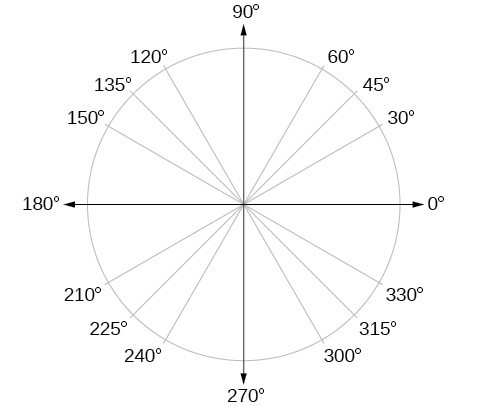Figure 14. Commonly encountered angles measured in degrees

Now, we can list the corresponding radian values for the common measures of a circle corresponding to those listed in Figure 14, which are shown in Figure 15. Be sure you can verify each of these measures.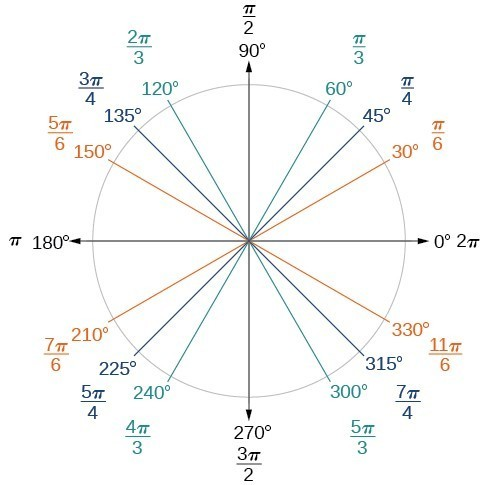Figure 15. Commonly encountered angles measured in radians

### EXAMPLE 2: FINDING A RADIAN MEASURE

Find the radian measure of one-third of a full rotation.

### TRY IT

Find the radian measure of three-fourths of a full rotation.

## Converting between Radians and Degrees

Because degrees and radians both measure angles, we need to be able to convert between them. We can easily do so using a proportion.

$\frac{\theta }{180}=\frac{{\theta }^{R}}{\pi }$

This proportion shows that the measure of angle $\theta$ in degrees divided by 180 equals the measure of angle $\theta$ in radians divided by $\pi .$ Or, phrased another way, degrees is to 180 as radians is to $\pi$.

$\frac{\text{Degrees}}{180}=\frac{\text{Radians}}{\pi }$

## Converting between Radians and Degrees

To convert between degrees and radians, use the proportion

$\frac{\theta }{180}=\frac{{\theta }^{R}}{\pi }$

### EXAMPLE 3: CONVERTING RADIANS TO DEGREES

Convert each radian measure to degrees.

a. $\frac{\pi }{6}$

b. 3

### TRY IT

Convert $-\frac{3\pi }{4}$ radians to degrees.

### EXAMPLE 4: CONVERTING DEGREES TO RADIANS

Convert $15$ degrees to radians.

### TRY IT

Convert 126° to radians.

### Try It

Watch the following video for an explanation of radian measure and examples of converting between radians and degrees.

### Degrees, Minutes, Seconds

A degree can be divided into 60 equal parts called minutes $(min')$ and each minute is divided into 60 equal parts called seconds $(sec'')$. Degrees, minutes, and seconds are written in the form $d^\circ m's''$. This has applications in navigation.

### HOW TO: Convert between decimal degrees and $d^\circ m's''$

1. To convert minutes into a degrees, multiply the minutes by $\frac{1^\circ}{60′}$
2. To convert seconds into a degrees, multiply the seconds by $\frac{1^\circ}{3600''}$
3. To convert a degree into a minute, multiply the degree by $\frac{60′}{1^\circ}$
4. To convert minutes into a seconds, multiply the minutes by $\frac{60''}{1′}$

### EXAMPLE 5: Converting $d^\circ m's''$ to Decimal Degrees

Convert $63^\circ 45'35''$ to decimal degrees. Round to four decimal places.

### EXAMPLE 6: Converting from decimal degrees to $d^\circ m's''$ form

Convert 47.6025° to $d^\circ m's''$ form.

### TRY IT

Convert $232^\circ 7'57''$ to decimal degrees.

### TRY IT

Convert $128.77^\circ$ to $d^\circ m's''$ form.

Recall that the radian measure $\theta$ of an angle was defined as the ratio of the arc length $s$ of a circular arc to the radius $r$ of the circle, $\theta =\frac{s}{r}$. From this relationship, we can find arc length along a circle, given an angle.

### A General Note: Arc Length on a Circle

In a circle of radius r, the length of an arc $s$ subtended by an angle with measure $\theta$ in radians, shown in Figure 20, is

$s=r\theta$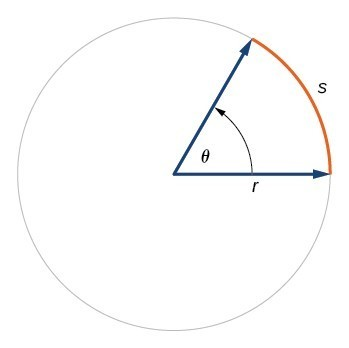Figure 20

### How To: Given a circle of radius $r$, calculate the length $s$ of the arc subtended by a given angle of measure $\theta$.

1. If necessary, convert $\theta$ to radians.
2. Multiply the radius $r$ by the radian measure of $\theta :s=r\theta$.

### Example 10: Finding the Length of an Arc

Assume the orbit of Mercury around the sun is a perfect circle. Mercury is approximately 36 million miles from the sun.

1. In one Earth day, Mercury completes 0.0114 of its total revolution. How many miles does it travel in one day?
2. Use your answer from part (a) to determine the radian measure for Mercury’s movement in one Earth day.

### Try It

Find the arc length along a circle of radius 10 units subtended by an angle of 215°.

### Try It

Finding the Area of a Sector of a Circle

In addition to arc length, we can also use angles to find the area of a sector of a circle. A sector is a region of a circle bounded by two radii and the intercepted arc, like a slice of pizza or pie. Recall that the area of a circle with radius $r$ can be found using the formula $A=\pi {r}^{2}$. If the two radii form an angle of $\theta$, measured in radians, then $\frac{\theta }{2\pi }$ is the ratio of the angle measure to the measure of a full rotation and is also, therefore, the ratio of the area of the sector to the area of the circle. Thus, the area of a sector is the fraction $\frac{\theta }{2\pi }$ multiplied by the entire area. (Always remember that this formula only applies if $\theta$ is in radians.)

\begin{align}\text{Area of sector}&=\left(\frac{\theta }{2\pi }\right)\pi {r}^{2} \\ &=\frac{\theta \pi {r}^{2}}{2\pi } \\ &=\frac{1}{2}\theta {r}^{2} \end{align}

### A General Note: Area of a Sector

The area of a sector of a circle with radius $r$ subtended by an angle $\theta$, measured in radians, is

$A=\frac{1}{2}\theta {r}^{2}$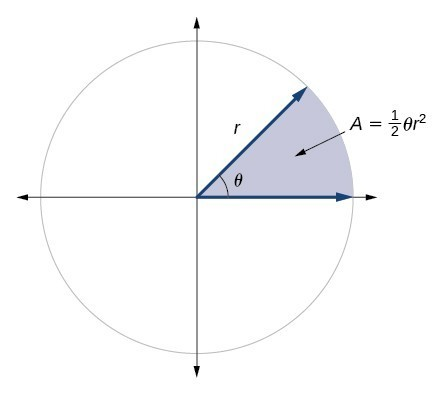Figure 21. The area of the sector equals half the square of the radius times the central angle measured in radians.

### How To: Given a circle of radius $r$, find the area of a sector defined by a given angle $\theta$.

1. If necessary, convert $\theta$ to radians.
2. Multiply half the radian measure of $\theta$ by the square of the radius $r:\text{ } A=\frac{1}{2}\theta {r}^{2}$.

### Example 11: Finding the Area of a Sector

An automatic lawn sprinkler sprays a distance of 20 feet while rotating 30 degrees, as shown in Figure 22. What is the area of the sector of grass the sprinkler waters?Figure 22. The sprinkler sprays 20 ft within an arc of 30°.

### Try It

In central pivot irrigation, a large irrigation pipe on wheels rotates around a center point. A farmer has a central pivot system with a radius of 400 meters. If water restrictions only allow her to water 150 thousand square meters a day, what angle should she set the system to cover? Write the answer in radian measure to two decimal places.

In the following video you will see how to calculate arc length and area of a sector of a circle.

## Use Linear and Angular Speed to Describe Motion on a Circular Path

In addition to finding the area of a sector, we can use angles to describe the speed of a moving object. An object traveling in a circular path has two types of speed. Linear speed is speed along a straight path and can be determined by the distance it moves along (its displacement) in a given time interval. For instance, if a wheel with radius 5 inches rotates once a second, a point on the edge of the wheel moves a distance equal to the circumference, or $10\pi$ inches, every second. So the linear speed of the point is $10\pi$ in./s. The equation for linear speed is as follows where $v$ is linear speed, $s$ is displacement, and $t$
is time.

$v=\frac{s}{t}$

Angular speed results from circular motion and can be determined by the angle through which a point rotates in a given time interval. In other words, angular speed is angular rotation per unit time. So, for instance, if a gear makes a full rotation every 4 seconds, we can calculate its angular speed as $\frac{360\text{ degrees}}{4\text{ seconds}}=$ 90 degrees per second. Angular speed can be given in radians per second, rotations per minute, or degrees per hour for example. The equation for angular speed is as follows, where $\omega$ (read as omega) is angular speed, $\theta$ is the angle traversed, and $t$ is time.

$\omega =\frac{\theta }{t}$

Combining the definition of angular speed with the arc length equation, $s=r\theta$, we can find a relationship between angular and linear speeds. The angular speed equation can be solved for $\theta$, giving $\theta =\omega t$. Substituting this into the arc length equation gives:

\begin{align}s&=r\theta \\ &=r\omega t \end{align}

Substituting this into the linear speed equation gives:

\begin{align} v&=\frac{s}{t} \\ &=\frac{r\omega t}{t} \\ &=r\omega \end{align}

### A General Note: Angular and Linear Speed

As a point moves along a circle of radius $r$, its angular speed, $\omega$, is the angular rotation $\theta$ per unit time, $t$.

$\omega =\frac{\theta }{t}$

The linear speed. $v$, of the point can be found as the distance traveled, arc length $s$, per unit time, $t$.

$v=\frac{s}{t}$

When the angular speed is measured in radians per unit time, linear speed and angular speed are related by the equation

$v=r\omega$

This equation states that the angular speed in radians, $\omega$, representing the amount of rotation occurring in a unit of time, can be multiplied by the radius $r$ to calculate the total arc length traveled in a unit of time, which is the definition of linear speed.

### How To: Given the amount of angle rotation and the time elapsed, calculate the angular speed.

1. If necessary, convert the angle measure to radians.
2. Divide the angle in radians by the number of time units elapsed: $\omega =\frac{\theta }{t}$.
3. The resulting speed will be in radians per time unit.

### Example 12: Finding Angular Speed

A water wheel, shown in Figure 23, completes 1 rotation every 5 seconds. Find the angular speed in radians per second.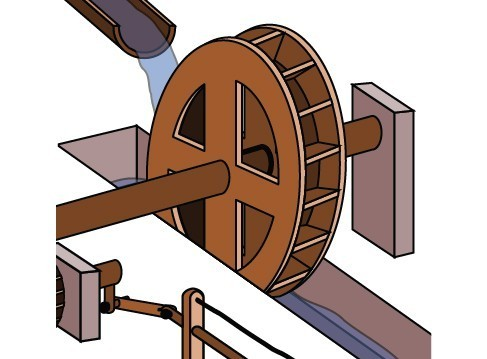Figure 23

### Try It

An old vinyl record is played on a turntable rotating clockwise at a rate of 45 rotations per minute. Find the angular speed in radians per second.

### How To: Given the radius of a circle, an angle of rotation, and a length of elapsed time, determine the linear speed.

1. Convert the total rotation to radians if necessary.
2. Divide the total rotation in radians by the elapsed time to find the angular speed: apply $\omega =\frac{\theta }{t}$.
3. Multiply the angular speed by the length of the radius to find the linear speed, expressed in terms of the length unit used for the radius and the time unit used for the elapsed time: apply $v=r\mathrm{\omega}$.

### Example 13: Finding a Linear Speed

A bicycle has wheels 28 inches in diameter. A tachometer determines the wheels are rotating at 180 RPM (revolutions per minute). Find the speed the bicycle is traveling down the road.

### Try It

A satellite is rotating around Earth at 0.25 radians per hour at an altitude of 242 km above Earth. If the radius of Earth is 6378 kilometers, find the linear speed of the satellite in kilometers per hour.

## Key Equations

 arc length $s=r\theta$ area of a sector $A=\frac{1}{2}\theta {r}^{2}$ angular speed $\omega =\frac{\theta }{t}$ linear speed $v=\frac{s}{t}$ linear speed related to angular speed $v=r\omega$

## Key Concepts

• An angle is formed from the union of two rays, by keeping the initial side fixed and rotating the terminal side. The amount of rotation determines the measure of the angle.
• An angle is in standard position if its vertex is at the origin and its initial side lies along the positive x-axis. A positive angle is measured counterclockwise from the initial side and a negative angle is measured clockwise.
• To draw an angle in standard position, draw the initial side along the positive x-axis and then place the terminal side according to the fraction of a full rotation the angle represents.
• In addition to degrees, the measure of an angle can be described in radians.
• To convert between degrees and radians, use the proportion $\frac{\theta }{180}=\frac{{\theta }^{R}}{\pi }$.
• Two angles that have the same terminal side are called coterminal angles.
• We can find coterminal angles by adding or subtracting 360° or $2\pi$.
• Coterminal angles can be found using radians just as they are for degrees.
• The length of a circular arc is a fraction of the circumference of the entire circle.
• The area of sector is a fraction of the area of the entire circle.
• An object moving in a circular path has both linear and angular speed.
• The angular speed of an object traveling in a circular path is the measure of the angle through which it turns in a unit of time.
• The linear speed of an object traveling along a circular path is the distance it travels in a unit of time.

## Glossary

angle
the union of two rays having a common endpoint
angular speed
the angle through which a rotating object travels in a unit of time
arc length
the length of the curve formed by an arc
area of a sector
area of a portion of a circle bordered by two radii and the intercepted arc; the fraction $\frac{\theta }{2\pi }$ multiplied by the area of the entire circle
coterminal angles
description of positive and negative angles in standard position sharing the same terminal side
degree
a unit of measure describing the size of an angle as one-360th of a full revolution of a circle
initial side
the side of an angle from which rotation begins
linear speed
the distance along a straight path a rotating object travels in a unit of time; determined by the arc length
measure of an angle
the amount of rotation from the initial side to the terminal side
negative angle
description of an angle measured clockwise from the positive x-axis
positive angle
description of an angle measured counterclockwise from the positive x-axis
quadrantal angle
an angle whose terminal side lies on an axis
radian measure
the ratio of the arc length formed by an angle divided by the radius of the circle
radian
the measure of a central angle of a circle that intercepts an arc equal in length to the radius of that circle
ray
one point on a line and all points extending in one direction from that point; one side of an angle
reference angle
the measure of the acute angle formed by the terminal side of the angle and the horizontal axis
standard position
the position of an angle having the vertex at the origin and the initial side along the positive x-axis
terminal side
the side of an angle at which rotation ends
vertex
the common endpoint of two rays that form an angle

## Section 4.1 Homework Exercises

1. Draw an angle in standard position. Label the vertex, initial side, and terminal side.

2. Explain why there are an infinite number of angles that are coterminal to a certain angle.

3. State what a positive or negative angle signifies, and explain how to draw each.

4. How does radian measure of an angle compare to the degree measure? Include an explanation of 1 radian in your paragraph.

5. Explain the differences between linear speed and angular speed when describing motion along a circular path.

For the following exercises, draw an angle in standard position with the given measure.

6. 30°

7. 300°

8. −80°

9. 135°

10. −150°

11. $\frac{2\pi }{3}$

12. $\frac{7\pi }{4}$

13. $\frac{5\pi }{6}$

14. $\frac{\pi }{2}$

15. $-\frac{\pi }{10}$

16. 415°

17. −120°

18. −315°

19. $\frac{22\pi }{3}$

20. $-\frac{\pi }{6}$

21. $-\frac{4\pi }{3}$

For the following exercises, round to two decimal places.

22. Find the arc length of a circle with a radius of 3 inches and central angle of 140 degrees.

23. Find the area of the sector of a circle with a radius of 3 inches and central angle of 140 degrees.

24. Find the area of the sector of a circle with a radius of 4.5 cm and central angle of $\frac{2\pi}{5}$ radians.

25. Find the arc length of a circle with a radius of 4.5 cm and central angle of $\frac{2\pi}{5}$ radians.

For the following exercises, convert angles in radians to degrees.

26. $\frac{3\pi }{4}$ radians

27. $\frac{\pi }{9}$ radians

28. $-\frac{5\pi }{4}$ radians

29. $\frac{\pi }{3}$ radians

30. $-\frac{7\pi }{3}$ radians

31. $-\frac{5\pi }{12}$ radians

32. $\frac{11\pi }{6}$ radians

For the following exercises, convert angles in degrees to radians.

33. 90°

34. 100°

35. −540°

36. −120°

37. 180°

38. −315°

39. 150°

For the following problems, convert each decimal degree measure into degrees-minutes-seconds and each degrees-minutes-seconds into decimal degrees.  Round your answer to three decimal places if necessary.

40. $136^\circ 51′ 18''$

41. $154^\circ 47′ 42''$

42. $49^\circ 36′ 9''$

43. $31^\circ 46′ 12″$

44. $17.725^\circ$

45. $2.9225^\circ$

46. $95.8375^\circ$

47. $36.87^\circ$

For the following exercises, use to given information to find the length of a circular arc. Round to two decimal places.

48. Find the length of the arc of a circle of radius 12 inches subtended by a central angle of $\frac{\pi }{4}$ radians.

49. Find the length of the arc of a circle of radius 5.02 miles subtended by the central angle of $\frac{\pi }{3}$.

50. Find the length of the arc of a circle of diameter 14 meters subtended by the central angle of $\frac{5\pi }{6}$.

51. Find the length of the arc of a circle of radius 10 centimeters subtended by the central angle of 50°.

52. Find the length of the arc of a circle of radius 5 inches subtended by the central angle of 220°.

53. Find the length of the arc of a circle of diameter 12 meters subtended by the central angle is 63°.

For the following exercises, use the given information to find the area of the sector. Round to four decimal places.

54. A sector of a circle has a central angle of 45° and a radius 6 cm.

55. A sector of a circle has a central angle of 30° and a radius of 20 cm.

56. A sector of a circle with diameter 10 feet and an angle of $\frac{\pi }{2}$ radians.

57. A sector of a circle with radius of 0.7 inches and an angle of $\pi$ radians.

For the following exercises, find the angle between 0° and 360° that is coterminal to the given angle.

58. −40°

59. −110°

60. 700°

61. 1400°

For the following exercises, find the angle between 0 and $2\pi$ in radians that is coterminal to the given angle.

62. $-\frac{\pi }{9}$

63. $\frac{10\pi }{3}$

64. $\frac{13\pi }{6}$

65. $\frac{44\pi }{9}$

66. A truck with 32-inch diameter wheels is traveling at 60 mi/h. Find the angular speed of the wheels in rad/min. How many revolutions per minute do the wheels make?

67. A bicycle with 24-inch diameter wheels is traveling at 15 mi/h. Find the angular speed of the wheels in rad/min. How many revolutions per minute do the wheels make?

68. A wheel of radius 8 inches is rotating 15°/s. What is the linear speed $v$, the angular speed in RPM, and the angular speed in rad/s?

69. A wheel of radius 14 inches is rotating 0.5 rad/s. What is the linear speed $v$, the angular speed in RPM, and the angular speed in deg/s?

70. A CD has diameter of 120 millimeters. When playing audio, the angular speed varies to keep the linear speed constant where the disc is being read. When reading along the outer edge of the disc, the angular speed is about 200 RPM (revolutions per minute). Find the linear speed.

71. When being burned in a writable CD-R drive, the angular speed of a CD is often much faster than when playing audio, but the angular speed still varies to keep the linear speed constant where the disc is being written. When writing along the outer edge of the disc, the angular speed of one drive is about 4800 RPM (revolutions per minute). Find the linear speed if the CD has diameter of 120 millimeters.

72. A person is standing on the equator of Earth (radius 3960 miles). What are his linear and angular speeds?

73. Find the distance along an arc on the surface of Earth that subtends a central angle of 5 minutes $\left(1\text{ minute}=\frac{1}{60}\text{ degree}\right)$ . The radius of Earth is 3960 miles.

74. Find the distance along an arc on the surface of Earth that subtends a central angle of 7 minutes $\left(1\text{ minute}=\frac{1}{60}\text{ degree}\right)$. The radius of Earth is $3960$ miles.

75. Consider a clock with an hour hand and minute hand. What is the measure of the angle the minute hand traces in $20$ minutes?

76. Two cities have the same longitude. The latitude of city A is 9.00 degrees north and the latitude of city B is 30.00 degree north. Assume the radius of the earth is 3960 miles. Find the distance between the two cities.

77. A city is located at 40 degrees north latitude. Assume the radius of the earth is 3960 miles and the earth rotates once every 24 hours. Find the linear speed of a person who resides in this city.

78. A city is located at 75 degrees north latitude. Assume the radius of the earth is 3960 miles and the earth rotates once every 24 hours. Find the linear speed of a person who resides in this city.

79. Find the linear speed of the moon if the average distance between the earth and moon is 239,000 miles, assuming the orbit of the moon is circular and requires about 28 days. Express answer in miles per hour.

80. A bicycle has wheels 28 inches in diameter. A tachometer determines that the wheels are rotating at 180 RPM (revolutions per minute). Find the speed the bicycle is travelling down the road.

81. A car travels 3 miles. Its tires make 2640 revolutions. What is the radius of a tire in inches?

82. A wheel on a tractor has a 24-inch diameter. How many revolutions does the wheel make if the tractor travels 4 miles?